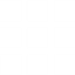# Welcome to Math CalculatorsMatrix Calculator
Matrix (plural matrices) is a rectangular array of numbers, symbols, or expressions, which is way to organize data in columns and rows.Volume Calculator
Calculate volume of geometric solids like Sphere, Rectangle, Square, Cube, Cone and Spherical Cap.Other Math CalculatorsArea Calculator
Calculate Area of Circle, Ellipse, Hexagonal Bar, Rectangle, Square, Triangle, Trapezoidal and many others.